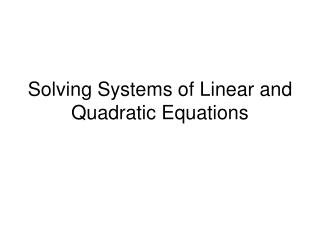DownloadDownload PresentationSolving Systems of Linear and Quadratic Equations

# Solving Systems of Linear and Quadratic Equations

Télécharger la présentation## Solving Systems of Linear and Quadratic Equations

- - - - - - - - - - - - - - - - - - - - - - - - - - - E N D - - - - - - - - - - - - - - - - - - - - - - - - - - -
##### Presentation Transcript

1. Solving Systems of Linear and Quadratic Equations

2. Remember these? Systems of Linear Equations We had 3 methods to solve them. Method 1 - Graphing Solve for y. (6, – 4)

3. Remember these? Systems of Linear Equations Method 2 - Substitution (6, – 4)

4. Remember these? Systems of Linear Equations Method 3 - Elimination All 3 methods giving us the same answer (6,–4). (6, – 4)

5. Now let’s look at Systems of Linear and Quadratic Equations!

6. Solve the System AlgebraicallyUse Substitution (0, 1) (1, 2) Answer: (0,1) (1,2)

7. Solve the System AlgebraicallyUse Substitution (2, 2) Answer: (2,2)

8. Solve the System AlgebraicallyUse Substitution (5, –1) (1, – 5) Answer: (5, –1) (1, – 5)

9. Solve the System AlgebraicallyUse Substitution (4, 3) (– 4, – 3) Answer: (4, 3) (–4, – 3)

10. Now let’s look at the Graphs of these Systems! Quadratic What does the graph of each look like? Parabola Classify each equation as linear/quadratic. Linear Line What is the solution to the system? Point of Intersection (-2, 0) Point of Intersection (1, -3 )

11. We have solved the following algebraically Now use your calculator to check it graphically. Answer: (0,1) (1,2) Answer: (2,2)

12. Equations must be solved for y!! Zoom 6: ZStandard The first equation can be entered as two separate entries: or a "list" notation may be used: Zoom 5: ZSquare Answer: (5, –1) (1, – 5)